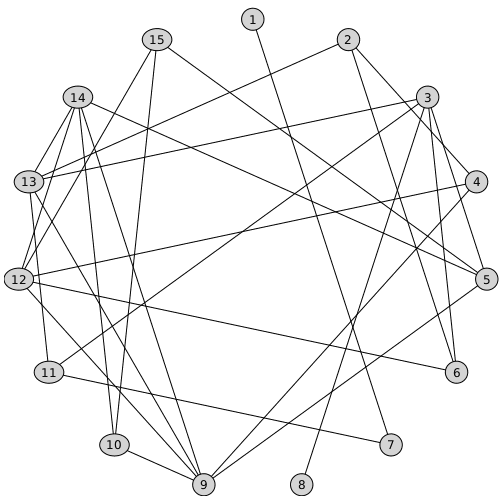SetVertexPositions - Maple Help

GraphTheory

 SetVertexPositionsCalling Sequence SetVertexPositions(G, vp)Parameters

 G - graph vp - list of pairs or triples of numbers, or numeric Matrix with 2 or 3 columnsDescription

 • SetVertexPositions('G', 'vp') sets the vertex positions vp of the graph G for graph drawing.  The vertex positions should be input in the format [[x1,y1],[x2,y2],...,[xn,yn]] or [[x1,y1,z1],[x2,y2,z2],...,[xn,yn,zn]].Examples

 > $\mathrm{with}\left(\mathrm{GraphTheory}\right):$
 > $G≔\mathrm{Graph}\left(6,\left\{\left\{1,2\right\},\left\{1,4\right\},\left\{4,5\right\},\left\{2,5\right\},\left\{2,3\right\},\left\{3,6\right\},\left\{5,6\right\}\right\}\right)$
 ${G}{≔}{\mathrm{Graph 1: an undirected graph with 6 vertices and 7 edge\left(s\right)}}$ (1)
 > $\mathrm{DrawGraph}\left(G\right)$> $\mathrm{vp}≔\left[\left[0,0\right],\left[0.5,0\right],\left[1,0\right],\left[0,0.5\right],\left[0.5,0.5\right],\left[1,0.5\right]\right]:$
 > $\mathrm{SetVertexPositions}\left(G,\mathrm{vp}\right):$
 > $\mathrm{DrawGraph}\left(G\right)$Compatibility

 • The GraphTheory[SetVertexPositions] command was updated in Maple 2020.